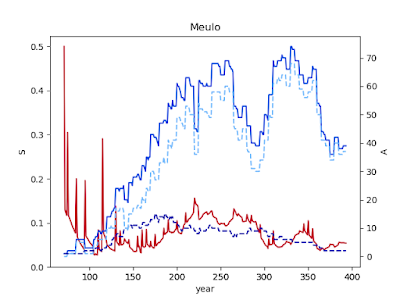## October 17, 2019

### History XX: Cliodynamics III

Continuing from here.

Recall these equations: $\dot{\log N} = r_0 \log N \left(1 - {\log N \over k_\textrm{max} - c W}\right) - d \log N W$ $\dot{\Xi} = \rho_0 \log N \left(1 - {\log N \over k_\textrm{max} - c W}\right) - b \log N$ $\dot{W} = a \log N^2 - b W - \alpha \Xi$, where $N$ is the population, $\Xi$ is the power of the state, and $W$ is the intensity of warfare.

Turchin's original works don't make it very clear that these are log-scaled numbers. I decided to track this discretely for each individual city, so I imagine there will be a handful of second-order effects.

This does result in some pretty intense cycling.$S$ in red, $A$ in blue
The total area fluctuates over about 200 years, while the $S$ lags that pattern slightly. I'm trying to think of a good way to tie $W$ or $\Xi$ to $S$, so that internal conflict will not arise when $S$ is high and collective solidarity is strong.

However, an error in the code (neglecting to restrict $k_\textrm{max} - c W$ to positive values) resulted in a situation where populations skyrocketed to billions of people in a single city.

Fixing that error introduced another artifact where it was extremely difficult for any significant hegemonies to arise and stabilize. The largest city topped out at less than 1500 inhabitants: barely a village.

The timescale is appropriate at about 200 years. But we want something more in terms of population, especially if we want the PCs to be able to have steel weapons and access to higher forms of magic such as wizardry and bardic training.

With some work, this could be tweaked to account for the longer lifespans of different races, if that's a thing in your game. If your elves live to 1000, their empires are more likely to last around 2000 years instead.